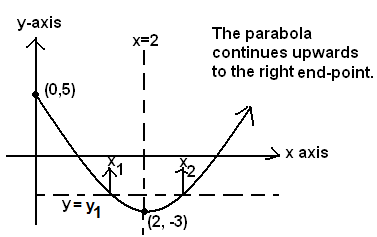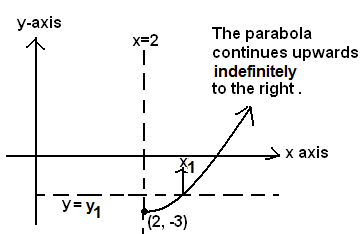## Monday, February 14, 2011

### An Add Maths Function Question

1 a) Express 2x2 – 8x + 5 in the form a(x + b)2 + c where a, b and c are integers. CIE ADD MATHS summer 2002, paper 1, question 11

Recall that (x + b) 2 = x 2 + 2bx + b 2. A common mistake is (x + b) 2 = x2 + b 2.  As a simple example, suppose x = b = 1. Then (1 + 1) 21 2 + 1 2.

Multiply (x + b) 2 through by a and add c: a(x + b) 2+ c = ax 2 + 2abx + ab 2 + c.

By polynomial identity, the squared terms of both forms can be equated, likewise the linear terms are equal, and finally the constant terms are also equal:

2x2 = ax 2     -->     a = 2
– 8x = 2abx     --> b = -2
5 = ab 2 + c    -->  c = -3

Thus 2x2 – 8x + 5  = 2(x + (-2))2 + (-3)

This sort of problem can also be solved by “completing the square,” which will be the subject of another blog. The equation y = 2x2 – 8x + 5 is written in expanded form, while y = 2(x – 2) 2 – 3 is the same parabola written in vertex form. As the squared term (x – 2) 2 cannot be negative, its minimum is 0 and this occurs when x = 2. Then, y = 2(0) 2 – 3 = -3. More generally a parabola when written as y = a(x – h) 2+ k has vertex (h, k). As an aside, the CIE examiner is deliberately muddying the waters by using the parameters a, b, and c in his writing of the vertex form. These particular letters are more commonly associated with the expanded form and its accompanying quadratic formula.

b) The function f is defined by f : x 2x2 – 8x + 5 for the domain 0 ≤ x ≤ 5.
i) Find the range of f.

From the expanded form (ax 2+bx +c) the y-intercept of the parabola is 5, the parabola is open
upwards (as a = 2 > 0) and from the vertex form (a(x - h)2 + k) its vertex is (2, -3). A rough sketch follows:The minimum y-value is -3. The left end-point maximum is y = 5. The right endpoint of the domain is x = 5 and the corresponding value of y is 2(5– 2) 2 – 3 = 18 – 3 = 15. Thus the range of function f is -3 <= y <=15.

ii) Explain why f does not have an inverse.

Notice the horizontal line y = y1. For one value of y there exist two values of x. The function is not 1-1. Therefore it cannot have an inverse.

The function g is defined by g : x 2x2– 8x + 5 for the domain x k.

iii) Find the smallest value of k for which g has an inverse.As seen above the graph of g should begin at the vertex and go upwards and right indefinitely. The domain is 2 ≤ x < infinity . Hence k = 2.

iv) For this value of k, find an expression for g–1

g(x) = y = 2x2– 8x + 5    Given
x = 2y2– 8y + 5    To find the inverse, swap ‘x’ and ‘y’.
x = 2(y – 2) 2 – 3     Switch to the (already known) vertex form, for easier simplification.
x + 3 = 2(y – 2) 2
½(x+3) = (y – 2) 2
sqrt{½(x+3)} =  sqrt { (y – 2)2 }   Square-root both sides; on the right, cancel the square and the square-root.
2 + sqrt {½(x+3)} = y = g–1
g–1(k) = g–1(2) = 2 +sqrt { ½(2+3)}    We were asked to find an expression; there is no requirement to simplify.